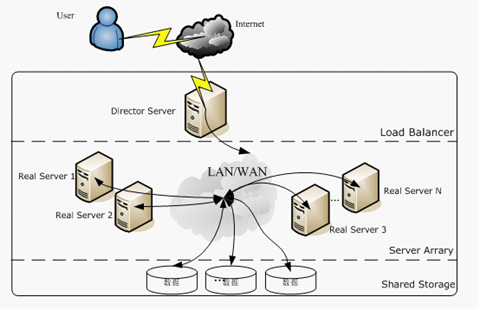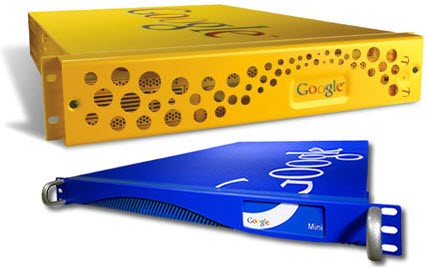项目 说明 PageRank 要抓取的资源的网页排名。 网页排名是影响资源在队列中位置的因素之一，它会让较重要文档的抓取频率高于较不重要的文档。 请注意，在导出快照时不会包含网页排名信息。 上次抓取时间 最后一次抓取该网址的时间。 下一个预定时间 预定抓取资源的时间。 该时间可能会改变，并且受网页排名、队列到期项目状况及其他因素的影响。过期项在被抓取前显示为红色。 如果您一直看到同一到期项，则可能需要进一步进行调查。 只有在首次抓取了网址之后，才会显示下一个预定时间。 更改间隔 希望对这一网络资源进行更改的频率。 首次抓取之后，设备将所有网址的更改间隔初始化为两天。 然后，设备会根据网址的实际更改频率调整更改间隔。 设备在每次抓取网址时都会了解在上次抓取后该资源是否发生过更改。 如果资源已更改，更改间隔会缩短。 如果资源未发生更改，更改间隔会延长。 您可以使用抓取频度调节功能更改这一计算过程。 只有在首次抓取了网址之后，“更改间隔”才会出现。 网址 所含内容被抓取的网络资源。

• PageRank会影响抓取频率
• 抓取频率受页面本身的更新频率影响

• 用户执行了哪些类型的查询？
• 用户点击了用户界面的哪些部分？
• 为用户提供服务的速度如何？
• 用户得到所需结果了吗？
• 您需要通过配置相关查询、关键字匹配、查询扩展或单一框功能帮助用户查找相关信息吗？

“高级搜索报告”中的每个条目表示用户在 Search Appliance 用户界面（搜索页或结果页）上的一次点击。 每个条目由以下项的值组成：

• 以百分之一秒为单位的点击时间
• 做出点击的用户的 IP 地址
• 会话 ID 的占据位置，始终为空
• 点击类型，如下表所述
• 点击起始页（用户点击得到的结果页）
• 点击排名（用户点击得到的结果页的排名）
• 点击数据，通常为空
• 查询（返回结果的用户查询）
• 用户点击的网址

 点击类型 说明 高级 搜索页上的高级搜索链接 advanced_swr 对其他文字的高级搜索 c 搜索结果 缓存 结果页上的缓存文档 集群 结果页上的集群标签 db 结果页上的数据库内容 desk.groups 搜索页顶部的论坛链接 desk.images 搜索页顶部的图片链接 desk.local 搜索页顶部的本地链接 desk.news 搜索页顶部的资讯链接 desk.web 搜索页顶部的网络链接 帮助 搜索页上的搜索提示链接 关键字匹配 结果页上的关键字匹配 徽标 超链接徽标 nav.next 导航，下一页 nav.page 导航，特定页 nav.prev 导航，上一页 单一框 结果页上的单一框 sitesearch 结果页上的“更多结果来自…链接” 排序 结果页上的排序链接 拼写 拼写建议 同义词 结果页上的相关查询 其他 未注解链接

• 用户在搜索结果页的操作可能影响后续的排名

• 用户给出的词的同词干词。例如，如果用户查找“engineer”，Search Appliance 能向查询中添加“engineers”。
• 一个或多个以空格分隔的字词（与用户提供的词互为同义词或与密切相关）。 例如，如果用户搜索“FAQ”，设备可能将“frequently asked questions”添加到该查询，或者如果用户输入“office building”，查询可能扩展到包含“office tower”。

• 部件制造商可以配置将作废部件号与替代部件号进行匹配的同义词。 对旧部件感兴趣的用户将也会收到新部件的相关信息。
• 大学可以通过配置同义词将课程缩写扩展成全称。 例如，关于 CS101 的查询可以包含从 computer science 101 得到的结果。
• 制造商可以配置其产品大类的相关查询，以便加入其产品名的查询。

• 做关键词优化前吗，需要确定是否有扩展关键词

• 域 – 将搜索限制在一个或多个域名（并非 IP 地址）
• 语言 – 将搜索限制在所有语言或所选择的一组语言
• 文件类型 – 将搜索限制在一个或多个文件类型，例如 HTML、PDF 等等
• 元标记 – 按元标记中的值和值类型过滤搜索，您可以通过元标记过滤搜索结果，通过使用“all”或“any”、值类型和值做出限制。
• 查询扩展策略 – 选择这样一种策略，该策略决定了利用同义词时查询所扩展的程度
• 结果自定义调整政策 – 选择一种影响 Search Appliance 评定文档与用户搜索查询
相关性方式的政策

 前端值类型 输入参数 精确 requiredfields=name:value 部分 partialfields=name:value 存在 requiredfields=name

• HTML页面标识语言
• 优先精确匹配

 搜索字词 相关查询 NATO 北大西洋公约组织 北大西洋公约组织 NATO Mark Twain Sam Clemens Sam Clemens 无

• 相关查询是单向的

 关键字匹配类型 标准（不区分大小写） 如果搜索查询是“Abraham Lincoln” 关键字匹配的原因 关键字匹配 必须出现在查询中某一位置的字词。 关键字匹配 =“Abraham”和“Lincoln” 如果您的关键字匹配为“Abraham Lincoln”，则搜索查询必需同时包含“Abraham”和“Lincoln”才能触发该关键字匹配。 要获得“Abraham”或“Lincoln”的关键字匹配，请输入两个关键字匹配： 一个用于“Abraham”，另一个用于“Lincoln”。 词组匹配 出现在查询中任何位置的词组。 对于匹配的词组，所有字词都必须出现，并且字词的顺序应相同，中间没有插入的字词，还要与查询中的所有连字符匹配。 词组匹配 = “Abraham Lincoln”、“President Abraham Lincoln”、“Abraham Lincoln president”和“young Abraham Lincoln” 由于字词是按照您在搜索查询（“Abraham Lincoln”）中输入的顺序显示的，因此这些都是词组关键字匹配。由于“the Tall”将词组“Abraham Lincoln”中的两个词分开，因此“Abraham the Tall Lincoln”不是词组匹配。 完全匹配 词组应与查询完全匹配。 完全匹配 = “Abraham Lincoln” 只有“Abraham Lincoln”与查询是完全匹配。 “President Abraham Lincoln”和“Abraham Lincoln’s”不是完全匹配。

Search Appliance 的集合数量上限是 1500，超过这个集合数目可能导致服务失败。重置索引可解决此问题。

### 默认集合

• 完整索引（您可以自行选择是否对用户公开）
• 基于语言的网页，支持仅搜索特定语言的网页
• 元标记 – 支持仅搜索具有特定元标记名称或名称值对的网页

### 搜索集合

• 在集合“Development”和“Marketing”中搜索“产品”：http://www.google.cn/search?q=产品&output=xml&client=yoursite&site=(development)|(marketing)。对于“product”的搜索结果来自于 Development 或 Marketing 集合。

### 始终强制重新抓取

• If-Modified-Since的使用

### 网络服务器主机负载例外

• 对您想使用代理来抓取的网站不指定主机负载，这种情况下会使用主机负载上限
• 指定足够小的主机负载以不影响任何代理站点的性能

• 每行只允许有一个主机名条目
• 主机负载为零 (0) 并不会完全停止抓取，而只是将与主机的接触次数减少为每小时大约三次
• 您可以将负载系数指定为小数值。 例如，5、1 或 2.0

• 服务器性能会影响抓取效率

Search Appliance 可从文档的标题、正文、网址或元标记中提取日期，也可从 HTTP 服务器返回的最后修改日期中提取日期。 默认情况下，会在 HTTP 标头针对所有文档返回的最后修改日期字段中查找日期。 文档日期搜索还会在非 HTML 文件的正文中查找日期。

Search Appliance 可以提取以下范围内的日期：

• 开始日期： 1970 年 1 月 1 日
• 结束日期： 从现在起两天后

Search Appliance 能够识别大多数格式合理的日期。 不过，请不要使用仅提及年份（YY 或 YYYY）的格式，例如 2002。 对于格式为月年的日期，则会假定日期为当月第一天。目前，文档日期可以识别大多数 Latin-1 月份名称，但不能识别中文、日语或韩语的月份名称。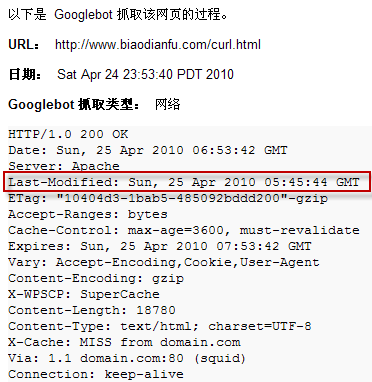• 网页的日期时间影响排名

### 抓取模式

Search Appliance 具有以下抓取模式：

• 持续抓取。 如果您想让抓取工具自动查找更新内容并编制索引，请选择该模式
• 预定抓取。 如果您想精确控制所有抓取的时间和持续时间，请选择该模式。 当出现以下情况时，预定抓取就会结束：
• 已经超过您指定的时间限制
• 抓取工具达到了您的许可指定的文档限制
• 抓取工具达到了“抓取并编制索引 > 主机负载计划”页上“抓取网址数量上限”下设置的限制
• 抓取工具已经抓取了所有可获得网址

### 抓取时间表

• 漫游器，主要针对的是所有网站，它会一直运行，主要是用来收集网址。
• 针对某一网站的限时抓取，所以怎么让搜索引擎在一定时间内索引到一定的页面就是SEO要做的内链的构建。同时搜索引擎一般在凌晨2点到4点之间大量抓取网页也是为了减轻目标服务器的压力。

# The following are popular filetype extensions - uncomment the lines to
# disable crawling them
# Microsoft Word
#regexpIgnoreCase:\\.doc$# Microsoft Excel #regexpIgnoreCase:\\.xls$
#regexpIgnoreCase:\\.xlw$# Microsoft Powerpoint #regexpIgnoreCase:\\.ppt$
# Microsoft Access
#regexpIgnoreCase:\\.mdb$# DBase / Xbase #regexpIgnoreCase:\\.dbf$
# Adobe Portable Document Format (PDF)
#regexpIgnoreCase:\\.pdf$# Rich Text Format (RTF) #regexpIgnoreCase:\\.rtf$
# The following are some typical Microsoft SharePoint patterns that you
# may not want to crawl - uncomment the lines to disable crawling them.
#contains:_layouts
#contains:_vti_bin
#WebFldr.aspx$#Upload.aspx$
#EditForm.aspx$# These patterns prevent crawling of repetitive URLs # prevents http://example.com/foo/foo/foo/..... regexp:/([^/]*)/\/\/ # prevents http://example.com/foo/bar/foo/bar/.... regexp:/([^/]*)/([^/]*)/\/\/ # prevents http://example.com/foo?bar=1&bar=1&bar=1... regexp:&([^&]*)&\&\ ############################################## # Filetypes we don't crawl # Images .gif$
.jpg$.jpeg$
.png$# Used instead of jpeg sometimes .jpe$
.pcx$.tif$
.tiff$.bmp$
# Binaries/Executables
regexpIgnoreCase:\\.dll$regexpIgnoreCase:\\.exe$
.a$.o$
.so$.bin$
.class$.jnilib$
# Font types
# true type font
.ttf$.pfb$
.pfm$.afm$
# Mac files
.hqx$.sea$
.dmg$# Adobe #.ps$
#.ps.gz$#.ps.Z$
.eps$.ai$
# Media
.ram$.wav$
.avi$.mid$
.mov$.mpg$
.mpeg$.mp3$
.ogg$.3gp$
.m4a$.m4v$
.wma$.wmv$
.wrl$# Databases .dat$
.dta$.log$
.lst$# Archives, except .ps.gz, .ps.Z .bz2$
.jar$.arj$
.cab$.rar$
.rpm$.tar$
.zip$.tar.gz$
.upp$.tgz$
.sdd$regexpIgnoreCase:([^.]..|[^p].|[^s])[.]z$
regexpIgnoreCase:([^.]..|[^p].|[^s])[.]gz$.lzh$
.msi$# Linux distribution files .hdr$
.iso$.img$
.gpg$# Google .gg$
.kml$.kmz$
.skb$.skp$
# Others
.gbk$.fac$
.ghg$.mdic$
.chm$.mht$
# Apache directory listings
/?S=A$/?S=D$
/?D=A$/?D=D$
/?M=A$/?M=D$
/?N=A$/?N=D$
/?C=N&O=A$/?C=M&O=A$
/?C=S&O=A$/?C=D&O=A$
/?C=N&O=D$/?C=M&O=D$
/?C=S&O=D$/?C=D&O=D$
/?C=N;O=A$/?C=M;O=A$
/?C=S;O=A$/?C=D;O=A$
/?C=N;O=D$/?C=M;O=D$
/?C=S;O=D$/?C=D;O=D$
# Invalid characters
contains:# The following are popular filetype extensions - uncomment the lines to
# disable crawling them
# Microsoft Word
#regexpIgnoreCase:\\.doc$# Microsoft Excel #regexpIgnoreCase:\\.xls$
#regexpIgnoreCase:\\.xlw$# Microsoft Powerpoint #regexpIgnoreCase:\\.ppt$
# Microsoft Access
#regexpIgnoreCase:\\.mdb$# DBase / Xbase #regexpIgnoreCase:\\.dbf$
# Adobe Portable Document Format (PDF)
#regexpIgnoreCase:\\.pdf$# Rich Text Format (RTF) #regexpIgnoreCase:\\.rtf$
# The following are some typical Microsoft SharePoint patterns that you
# may not want to crawl - uncomment the lines to disable crawling them.
#contains:_layouts
#contains:_vti_bin
#WebFldr.aspx$#Upload.aspx$
#EditForm.aspx$# These patterns prevent crawling of repetitive URLs # prevents http://example.com/foo/foo/foo/..... regexp:/([^/]*)/\\1/\\1/ # prevents http://example.com/foo/bar/foo/bar/.... regexp:/([^/]*)/([^/]*)/\\1/\\2/ # prevents http://example.com/foo?bar=1&bar=1&bar=1... regexp:&([^&]*)&\\1&\\1 ############################################## # Filetypes we don't crawl # Images .gif$
.jpg$.jpeg$
.png$# Used instead of jpeg sometimes .jpe$
.pcx$.tif$
.tiff$.bmp$
# Binaries/Executables
regexpIgnoreCase:\\.dll$regexpIgnoreCase:\\.exe$
.a$.o$
.so$.bin$
.class$.jnilib$
# Font types
# true type font
.ttf$.pfb$
.pfm$.afm$
# Mac files
.hqx$.sea$
.dmg$# Adobe #.ps$
#.ps.gz$#.ps.Z$
.eps$.ai$
# Media
.ram$.wav$
.avi$.mid$
.mov$.mpg$
.mpeg$.mp3$
.ogg$.3gp$
.m4a$.m4v$
.wma$.wmv$
.wrl$# Databases .dat$
.dta$.log$
.lst$# Archives, except .ps.gz, .ps.Z .bz2$
.jar$.arj$
.cab$.rar$
.rpm$.tar$
.zip$.tar.gz$
.upp$.tgz$
.sdd$regexpIgnoreCase:([^.]..|[^p].|[^s])[.]z$
regexpIgnoreCase:([^.]..|[^p].|[^s])[.]gz$.lzh$
.msi$# Linux distribution files .hdr$
.iso$.img$
.gpg$# Google .gg$
.kml$.kmz$
.skb$.skp$
# Others
.gbk$.fac$
.ghg$.mdic$
.chm$.mht$
# Apache directory listings
/?S=A$/?S=D$
/?D=A$/?D=D$
/?M=A$/?M=D$
/?N=A$/?N=D$
/?C=N&O=A$/?C=M&O=A$
/?C=S&O=A$/?C=D&O=A$
/?C=N&O=D$/?C=M&O=D$
/?C=S&O=D$/?C=D&O=D$
/?C=N;O=A$/?C=M;O=A$
/?C=S;O=A$/?C=D;O=A$
/?C=N;O=D$/?C=M;O=D$
/?C=S;O=D$/?C=D;O=D$
# Invalid characters
contains:\001
contains:\002
contains:\003
contains:\004
contains:\005
contains:\006
contains:\007
contains:\010
contains:\011
contains:\012
contains:\013
contains:\014
contains:\015
contains:\016
contains:\017
contains:\020
contains:\021
contains:\022
contains:\023
contains:\024
contains:\025
contains:\026
contains:\027
contains:\030
contains:\031
contains:\032
contains:\033
contains:\034
contains:\035
contains:\036
contains:\037
contains:\040
contains:\177
# Invalid endings
.html/$.htm/$
.phtml/$.ghtml/$
.asp/$.jsp/$
.shtml/$# Invalid endings !/ "/$/
%/
&/
'/
(/
)/
+/
,/
./
</
=/
>/
{/
|/
}/
~/
[/
\\\
]/
^/
/
1
contains:# The following are popular filetype extensions - uncomment the lines to
# disable crawling them
# Microsoft Word
#regexpIgnoreCase:\\.doc$# Microsoft Excel #regexpIgnoreCase:\\.xls$
#regexpIgnoreCase:\\.xlw$# Microsoft Powerpoint #regexpIgnoreCase:\\.ppt$
# Microsoft Access
#regexpIgnoreCase:\\.mdb$# DBase / Xbase #regexpIgnoreCase:\\.dbf$
# Adobe Portable Document Format (PDF)
#regexpIgnoreCase:\\.pdf$# Rich Text Format (RTF) #regexpIgnoreCase:\\.rtf$
# The following are some typical Microsoft SharePoint patterns that you
# may not want to crawl - uncomment the lines to disable crawling them.
#contains:_layouts
#contains:_vti_bin
#WebFldr.aspx$#Upload.aspx$
#EditForm.aspx$# These patterns prevent crawling of repetitive URLs # prevents http://example.com/foo/foo/foo/..... regexp:/([^/]*)/\\1/\\1/ # prevents http://example.com/foo/bar/foo/bar/.... regexp:/([^/]*)/([^/]*)/\\1/\\2/ # prevents http://example.com/foo?bar=1&bar=1&bar=1... regexp:&([^&]*)&\\1&\\1 ############################################## # Filetypes we don't crawl # Images .gif$
.jpg$.jpeg$
.png$# Used instead of jpeg sometimes .jpe$
.pcx$.tif$
.tiff$.bmp$
# Binaries/Executables
regexpIgnoreCase:\\.dll$regexpIgnoreCase:\\.exe$
.a$.o$
.so$.bin$
.class$.jnilib$
# Font types
# true type font
.ttf$.pfb$
.pfm$.afm$
# Mac files
.hqx$.sea$
.dmg$# Adobe #.ps$
#.ps.gz$#.ps.Z$
.eps$.ai$
# Media
.ram$.wav$
.avi$.mid$
.mov$.mpg$
.mpeg$.mp3$
.ogg$.3gp$
.m4a$.m4v$
.wma$.wmv$
.wrl$# Databases .dat$
.dta$.log$
.lst$# Archives, except .ps.gz, .ps.Z .bz2$
.jar$.arj$
.cab$.rar$
.rpm$.tar$
.zip$.tar.gz$
.upp$.tgz$
.sdd$regexpIgnoreCase:([^.]..|[^p].|[^s])[.]z$
regexpIgnoreCase:([^.]..|[^p].|[^s])[.]gz$.lzh$
.msi$# Linux distribution files .hdr$
.iso$.img$
.gpg$# Google .gg$
.kml$.kmz$
.skb$.skp$
# Others
.gbk$.fac$
.ghg$.mdic$
.chm$.mht$
# Apache directory listings
/?S=A$/?S=D$
/?D=A$/?D=D$
/?M=A$/?M=D$
/?N=A$/?N=D$
/?C=N&O=A$/?C=M&O=A$
/?C=S&O=A$/?C=D&O=A$
/?C=N&O=D$/?C=M&O=D$
/?C=S&O=D$/?C=D&O=D$
/?C=N;O=A$/?C=M;O=A$
/?C=S;O=A$/?C=D;O=A$
/?C=N;O=D$/?C=M;O=D$
/?C=S;O=D$/?C=D;O=D$
# Invalid characters
contains:\001
contains:\002
contains:\003
contains:\004
contains:\005
contains:\006
contains:\007
contains:\010
contains:\011
contains:\012
contains:\013
contains:\014
contains:\015
contains:\016
contains:\017
contains:\020
contains:\021
contains:\022
contains:\023
contains:\024
contains:\025
contains:\026
contains:\027
contains:\030
contains:\031
contains:\032
contains:\033
contains:\034
contains:\035
contains:\036
contains:\037
contains:\040
contains:\177
# Invalid endings
.html/$.htm/$
.phtml/$.ghtml/$
.asp/$.jsp/$
.shtml/$# Invalid endings !/ "/$/
%/
&/
'/
(/
)/
+/
,/
./
</
=/
>/
{/
|/
}/
~/
[/
\\\
]/
^/
/
2
contains:# The following are popular filetype extensions - uncomment the lines to
# disable crawling them
# Microsoft Word
#regexpIgnoreCase:\\.doc$# Microsoft Excel #regexpIgnoreCase:\\.xls$
#regexpIgnoreCase:\\.xlw$# Microsoft Powerpoint #regexpIgnoreCase:\\.ppt$
# Microsoft Access
#regexpIgnoreCase:\\.mdb$# DBase / Xbase #regexpIgnoreCase:\\.dbf$
# Adobe Portable Document Format (PDF)
#regexpIgnoreCase:\\.pdf$# Rich Text Format (RTF) #regexpIgnoreCase:\\.rtf$
# The following are some typical Microsoft SharePoint patterns that you
# may not want to crawl - uncomment the lines to disable crawling them.
#contains:_layouts
#contains:_vti_bin
#WebFldr.aspx$#Upload.aspx$
#EditForm.aspx$# These patterns prevent crawling of repetitive URLs # prevents http://example.com/foo/foo/foo/..... regexp:/([^/]*)/\\1/\\1/ # prevents http://example.com/foo/bar/foo/bar/.... regexp:/([^/]*)/([^/]*)/\\1/\\2/ # prevents http://example.com/foo?bar=1&bar=1&bar=1... regexp:&([^&]*)&\\1&\\1 ############################################## # Filetypes we don't crawl # Images .gif$
.jpg$.jpeg$
.png$# Used instead of jpeg sometimes .jpe$
.pcx$.tif$
.tiff$.bmp$
# Binaries/Executables
regexpIgnoreCase:\\.dll$regexpIgnoreCase:\\.exe$
.a$.o$
.so$.bin$
.class$.jnilib$
# Font types
# true type font
.ttf$.pfb$
.pfm$.afm$
# Mac files
.hqx$.sea$
.dmg$# Adobe #.ps$
#.ps.gz$#.ps.Z$
.eps$.ai$
# Media
.ram$.wav$
.avi$.mid$
.mov$.mpg$
.mpeg$.mp3$
.ogg$.3gp$
.m4a$.m4v$
.wma$.wmv$
.wrl$# Databases .dat$
.dta$.log$
.lst$# Archives, except .ps.gz, .ps.Z .bz2$
.jar$.arj$
.cab$.rar$
.rpm$.tar$
.zip$.tar.gz$
.upp$.tgz$
.sdd$regexpIgnoreCase:([^.]..|[^p].|[^s])[.]z$
regexpIgnoreCase:([^.]..|[^p].|[^s])[.]gz$.lzh$
.msi$# Linux distribution files .hdr$
.iso$.img$
.gpg$# Google .gg$
.kml$.kmz$
.skb$.skp$
# Others
.gbk$.fac$
.ghg$.mdic$
.chm$.mht$
# Apache directory listings
/?S=A$/?S=D$
/?D=A$/?D=D$
/?M=A$/?M=D$
/?N=A$/?N=D$
/?C=N&O=A$/?C=M&O=A$
/?C=S&O=A$/?C=D&O=A$
/?C=N&O=D$/?C=M&O=D$
/?C=S&O=D$/?C=D&O=D$
/?C=N;O=A$/?C=M;O=A$
/?C=S;O=A$/?C=D;O=A$
/?C=N;O=D$/?C=M;O=D$
/?C=S;O=D$/?C=D;O=D$
# Invalid characters
contains:\001
contains:\002
contains:\003
contains:\004
contains:\005
contains:\006
contains:\007
contains:\010
contains:\011
contains:\012
contains:\013
contains:\014
contains:\015
contains:\016
contains:\017
contains:\020
contains:\021
contains:\022
contains:\023
contains:\024
contains:\025
contains:\026
contains:\027
contains:\030
contains:\031
contains:\032
contains:\033
contains:\034
contains:\035
contains:\036
contains:\037
contains:\040
contains:\177
# Invalid endings
.html/$.htm/$
.phtml/$.ghtml/$
.asp/$.jsp/$
.shtml/$# Invalid endings !/ "/$/
%/
&/
'/
(/
)/
+/
,/
./
</
=/
>/
{/
|/
}/
~/
[/
\\\
]/
^/
/
3
contains:# The following are popular filetype extensions - uncomment the lines to
# disable crawling them
# Microsoft Word
#regexpIgnoreCase:\\.doc$# Microsoft Excel #regexpIgnoreCase:\\.xls$
#regexpIgnoreCase:\\.xlw$# Microsoft Powerpoint #regexpIgnoreCase:\\.ppt$
# Microsoft Access
#regexpIgnoreCase:\\.mdb$# DBase / Xbase #regexpIgnoreCase:\\.dbf$
# Adobe Portable Document Format (PDF)
#regexpIgnoreCase:\\.pdf$# Rich Text Format (RTF) #regexpIgnoreCase:\\.rtf$
# The following are some typical Microsoft SharePoint patterns that you
# may not want to crawl - uncomment the lines to disable crawling them.
#contains:_layouts
#contains:_vti_bin
#WebFldr.aspx$#Upload.aspx$
#EditForm.aspx$# These patterns prevent crawling of repetitive URLs # prevents http://example.com/foo/foo/foo/..... regexp:/([^/]*)/\\1/\\1/ # prevents http://example.com/foo/bar/foo/bar/.... regexp:/([^/]*)/([^/]*)/\\1/\\2/ # prevents http://example.com/foo?bar=1&bar=1&bar=1... regexp:&([^&]*)&\\1&\\1 ############################################## # Filetypes we don't crawl # Images .gif$
.jpg$.jpeg$
.png$# Used instead of jpeg sometimes .jpe$
.pcx$.tif$
.tiff$.bmp$
# Binaries/Executables
regexpIgnoreCase:\\.dll$regexpIgnoreCase:\\.exe$
.a$.o$
.so$.bin$
.class$.jnilib$
# Font types
# true type font
.ttf$.pfb$
.pfm$.afm$
# Mac files
.hqx$.sea$
.dmg$# Adobe #.ps$
#.ps.gz$#.ps.Z$
.eps$.ai$
# Media
.ram$.wav$
.avi$.mid$
.mov$.mpg$
.mpeg$.mp3$
.ogg$.3gp$
.m4a$.m4v$
.wma$.wmv$
.wrl$# Databases .dat$
.dta$.log$
.lst$# Archives, except .ps.gz, .ps.Z .bz2$
.jar$.arj$
.cab$.rar$
.rpm$.tar$
.zip$.tar.gz$
.upp$.tgz$
.sdd$regexpIgnoreCase:([^.]..|[^p].|[^s])[.]z$
regexpIgnoreCase:([^.]..|[^p].|[^s])[.]gz$.lzh$
.msi$# Linux distribution files .hdr$
.iso$.img$
.gpg$# Google .gg$
.kml$.kmz$
.skb$.skp$
# Others
.gbk$.fac$
.ghg$.mdic$
.chm$.mht$
# Apache directory listings
/?S=A$/?S=D$
/?D=A$/?D=D$
/?M=A$/?M=D$
/?N=A$/?N=D$
/?C=N&O=A$/?C=M&O=A$
/?C=S&O=A$/?C=D&O=A$
/?C=N&O=D$/?C=M&O=D$
/?C=S&O=D$/?C=D&O=D$
/?C=N;O=A$/?C=M;O=A$
/?C=S;O=A$/?C=D;O=A$
/?C=N;O=D$/?C=M;O=D$
/?C=S;O=D$/?C=D;O=D$
# Invalid characters
contains:\001
contains:\002
contains:\003
contains:\004
contains:\005
contains:\006
contains:\007
contains:\010
contains:\011
contains:\012
contains:\013
contains:\014
contains:\015
contains:\016
contains:\017
contains:\020
contains:\021
contains:\022
contains:\023
contains:\024
contains:\025
contains:\026
contains:\027
contains:\030
contains:\031
contains:\032
contains:\033
contains:\034
contains:\035
contains:\036
contains:\037
contains:\040
contains:\177
# Invalid endings
.html/$.htm/$
.phtml/$.ghtml/$
.asp/$.jsp/$
.shtml/$# Invalid endings !/ "/$/
%/
&/
'/
(/
)/
+/
,/
./
</
=/
>/
{/
|/
}/
~/
[/
\\\
]/
^/
/
4
contains:# The following are popular filetype extensions - uncomment the lines to
# disable crawling them
# Microsoft Word
#regexpIgnoreCase:\\.doc$# Microsoft Excel #regexpIgnoreCase:\\.xls$
#regexpIgnoreCase:\\.xlw$# Microsoft Powerpoint #regexpIgnoreCase:\\.ppt$
# Microsoft Access
#regexpIgnoreCase:\\.mdb$# DBase / Xbase #regexpIgnoreCase:\\.dbf$
# Adobe Portable Document Format (PDF)
#regexpIgnoreCase:\\.pdf$# Rich Text Format (RTF) #regexpIgnoreCase:\\.rtf$
# The following are some typical Microsoft SharePoint patterns that you
# may not want to crawl - uncomment the lines to disable crawling them.
#contains:_layouts
#contains:_vti_bin
#WebFldr.aspx$#Upload.aspx$
#EditForm.aspx$# These patterns prevent crawling of repetitive URLs # prevents http://example.com/foo/foo/foo/..... regexp:/([^/]*)/\\1/\\1/ # prevents http://example.com/foo/bar/foo/bar/.... regexp:/([^/]*)/([^/]*)/\\1/\\2/ # prevents http://example.com/foo?bar=1&bar=1&bar=1... regexp:&([^&]*)&\\1&\\1 ############################################## # Filetypes we don't crawl # Images .gif$
.jpg$.jpeg$
.png$# Used instead of jpeg sometimes .jpe$
.pcx$.tif$
.tiff$.bmp$
# Binaries/Executables
regexpIgnoreCase:\\.dll$regexpIgnoreCase:\\.exe$
.a$.o$
.so$.bin$
.class$.jnilib$
# Font types
# true type font
.ttf$.pfb$
.pfm$.afm$
# Mac files
.hqx$.sea$
.dmg$# Adobe #.ps$
#.ps.gz$#.ps.Z$
.eps$.ai$
# Media
.ram$.wav$
.avi$.mid$
.mov$.mpg$
.mpeg$.mp3$
.ogg$.3gp$
.m4a$.m4v$
.wma$.wmv$
.wrl$# Databases .dat$
.dta$.log$
.lst$# Archives, except .ps.gz, .ps.Z .bz2$
.jar$.arj$
.cab$.rar$
.rpm$.tar$
.zip$.tar.gz$
.upp$.tgz$
.sdd$regexpIgnoreCase:([^.]..|[^p].|[^s])[.]z$
regexpIgnoreCase:([^.]..|[^p].|[^s])[.]gz$.lzh$
.msi$# Linux distribution files .hdr$
.iso$.img$
.gpg$# Google .gg$
.kml$.kmz$
.skb$.skp$
# Others
.gbk$.fac$
.ghg$.mdic$
.chm$.mht$
# Apache directory listings
/?S=A$/?S=D$
/?D=A$/?D=D$
/?M=A$/?M=D$
/?N=A$/?N=D$
/?C=N&O=A$/?C=M&O=A$
/?C=S&O=A$/?C=D&O=A$
/?C=N&O=D$/?C=M&O=D$
/?C=S&O=D$/?C=D&O=D$
/?C=N;O=A$/?C=M;O=A$
/?C=S;O=A$/?C=D;O=A$
/?C=N;O=D$/?C=M;O=D$
/?C=S;O=D$/?C=D;O=D$
# Invalid characters
contains:\001
contains:\002
contains:\003
contains:\004
contains:\005
contains:\006
contains:\007
contains:\010
contains:\011
contains:\012
contains:\013
contains:\014
contains:\015
contains:\016
contains:\017
contains:\020
contains:\021
contains:\022
contains:\023
contains:\024
contains:\025
contains:\026
contains:\027
contains:\030
contains:\031
contains:\032
contains:\033
contains:\034
contains:\035
contains:\036
contains:\037
contains:\040
contains:\177
# Invalid endings
.html/$.htm/$
.phtml/$.ghtml/$
.asp/$.jsp/$
.shtml/$# Invalid endings !/ "/$/
%/
&/
'/
(/
)/
+/
,/
./
</
=/
>/
{/
|/
}/
~/
[/
\\\
]/
^/
/
5
contains:# The following are popular filetype extensions - uncomment the lines to
# disable crawling them
# Microsoft Word
#regexpIgnoreCase:\\.doc$# Microsoft Excel #regexpIgnoreCase:\\.xls$
#regexpIgnoreCase:\\.xlw$# Microsoft Powerpoint #regexpIgnoreCase:\\.ppt$
# Microsoft Access
#regexpIgnoreCase:\\.mdb$# DBase / Xbase #regexpIgnoreCase:\\.dbf$
# Adobe Portable Document Format (PDF)
#regexpIgnoreCase:\\.pdf$# Rich Text Format (RTF) #regexpIgnoreCase:\\.rtf$
# The following are some typical Microsoft SharePoint patterns that you
# may not want to crawl - uncomment the lines to disable crawling them.
#contains:_layouts
#contains:_vti_bin
#WebFldr.aspx$#Upload.aspx$
#EditForm.aspx$# These patterns prevent crawling of repetitive URLs # prevents http://example.com/foo/foo/foo/..... regexp:/([^/]*)/\\1/\\1/ # prevents http://example.com/foo/bar/foo/bar/.... regexp:/([^/]*)/([^/]*)/\\1/\\2/ # prevents http://example.com/foo?bar=1&bar=1&bar=1... regexp:&([^&]*)&\\1&\\1 ############################################## # Filetypes we don't crawl # Images .gif$
.jpg$.jpeg$
.png$# Used instead of jpeg sometimes .jpe$
.pcx$.tif$
.tiff$.bmp$
# Binaries/Executables
regexpIgnoreCase:\\.dll$regexpIgnoreCase:\\.exe$
.a$.o$
.so$.bin$
.class$.jnilib$
# Font types
# true type font
.ttf$.pfb$
.pfm$.afm$
# Mac files
.hqx$.sea$
.dmg$# Adobe #.ps$
#.ps.gz$#.ps.Z$
.eps$.ai$
# Media
.ram$.wav$
.avi$.mid$
.mov$.mpg$
.mpeg$.mp3$
.ogg$.3gp$
.m4a$.m4v$
.wma$.wmv$
.wrl$# Databases .dat$
.dta$.log$
.lst$# Archives, except .ps.gz, .ps.Z .bz2$
.jar$.arj$
.cab$.rar$
.rpm$.tar$
.zip$.tar.gz$
.upp$.tgz$
.sdd$regexpIgnoreCase:([^.]..|[^p].|[^s])[.]z$
regexpIgnoreCase:([^.]..|[^p].|[^s])[.]gz$.lzh$
.msi$# Linux distribution files .hdr$
.iso$.img$
.gpg$# Google .gg$
.kml$.kmz$
.skb$.skp$
# Others
.gbk$.fac$
.ghg$.mdic$
.chm$.mht$
# Apache directory listings
/?S=A$/?S=D$
/?D=A$/?D=D$
/?M=A$/?M=D$
/?N=A$/?N=D$
/?C=N&O=A$/?C=M&O=A$
/?C=S&O=A$/?C=D&O=A$
/?C=N&O=D$/?C=M&O=D$
/?C=S&O=D$/?C=D&O=D$
/?C=N;O=A$/?C=M;O=A$
/?C=S;O=A$/?C=D;O=A$
/?C=N;O=D$/?C=M;O=D$
/?C=S;O=D$/?C=D;O=D$
# Invalid characters
contains:\001
contains:\002
contains:\003
contains:\004
contains:\005
contains:\006
contains:\007
contains:\010
contains:\011
contains:\012
contains:\013
contains:\014
contains:\015
contains:\016
contains:\017
contains:\020
contains:\021
contains:\022
contains:\023
contains:\024
contains:\025
contains:\026
contains:\027
contains:\030
contains:\031
contains:\032
contains:\033
contains:\034
contains:\035
contains:\036
contains:\037
contains:\040
contains:\177
# Invalid endings
.html/$.htm/$
.phtml/$.ghtml/$
.asp/$.jsp/$
.shtml/$# Invalid endings !/ "/$/
%/
&/
'/
(/
)/
+/
,/
./
</
=/
>/
{/
|/
}/
~/
[/
\\\
]/
^/
/
6
contains:# The following are popular filetype extensions - uncomment the lines to
# disable crawling them
# Microsoft Word
#regexpIgnoreCase:\\.doc$# Microsoft Excel #regexpIgnoreCase:\\.xls$
#regexpIgnoreCase:\\.xlw$# Microsoft Powerpoint #regexpIgnoreCase:\\.ppt$
# Microsoft Access
#regexpIgnoreCase:\\.mdb$# DBase / Xbase #regexpIgnoreCase:\\.dbf$
# Adobe Portable Document Format (PDF)
#regexpIgnoreCase:\\.pdf$# Rich Text Format (RTF) #regexpIgnoreCase:\\.rtf$
# The following are some typical Microsoft SharePoint patterns that you
# may not want to crawl - uncomment the lines to disable crawling them.
#contains:_layouts
#contains:_vti_bin
#WebFldr.aspx$#Upload.aspx$
#EditForm.aspx$# These patterns prevent crawling of repetitive URLs # prevents http://example.com/foo/foo/foo/..... regexp:/([^/]*)/\\1/\\1/ # prevents http://example.com/foo/bar/foo/bar/.... regexp:/([^/]*)/([^/]*)/\\1/\\2/ # prevents http://example.com/foo?bar=1&bar=1&bar=1... regexp:&([^&]*)&\\1&\\1 ############################################## # Filetypes we don't crawl # Images .gif$
.jpg$.jpeg$
.png$# Used instead of jpeg sometimes .jpe$
.pcx$.tif$
.tiff$.bmp$
# Binaries/Executables
regexpIgnoreCase:\\.dll$regexpIgnoreCase:\\.exe$
.a$.o$
.so$.bin$
.class$.jnilib$
# Font types
# true type font
.ttf$.pfb$
.pfm$.afm$
# Mac files
.hqx$.sea$
.dmg$# Adobe #.ps$
#.ps.gz$#.ps.Z$
.eps$.ai$
# Media
.ram$.wav$
.avi$.mid$
.mov$.mpg$
.mpeg$.mp3$
.ogg$.3gp$
.m4a$.m4v$
.wma$.wmv$
.wrl$# Databases .dat$
.dta$.log$
.lst$# Archives, except .ps.gz, .ps.Z .bz2$
.jar$.arj$
.cab$.rar$
.rpm$.tar$
.zip$.tar.gz$
.upp$.tgz$
.sdd$regexpIgnoreCase:([^.]..|[^p].|[^s])[.]z$
regexpIgnoreCase:([^.]..|[^p].|[^s])[.]gz$.lzh$
.msi$# Linux distribution files .hdr$
.iso$.img$
.gpg$# Google .gg$
.kml$.kmz$
.skb$.skp$
# Others
.gbk$.fac$
.ghg$.mdic$
.chm$.mht$
# Apache directory listings
/?S=A$/?S=D$
/?D=A$/?D=D$
/?M=A$/?M=D$
/?N=A$/?N=D$
/?C=N&O=A$/?C=M&O=A$
/?C=S&O=A$/?C=D&O=A$
/?C=N&O=D$/?C=M&O=D$
/?C=S&O=D$/?C=D&O=D$
/?C=N;O=A$/?C=M;O=A$
/?C=S;O=A$/?C=D;O=A$
/?C=N;O=D$/?C=M;O=D$
/?C=S;O=D$/?C=D;O=D$
# Invalid characters
contains:\001
contains:\002
contains:\003
contains:\004
contains:\005
contains:\006
contains:\007
contains:\010
contains:\011
contains:\012
contains:\013
contains:\014
contains:\015
contains:\016
contains:\017
contains:\020
contains:\021
contains:\022
contains:\023
contains:\024
contains:\025
contains:\026
contains:\027
contains:\030
contains:\031
contains:\032
contains:\033
contains:\034
contains:\035
contains:\036
contains:\037
contains:\040
contains:\177
# Invalid endings
.html/$.htm/$
.phtml/$.ghtml/$
.asp/$.jsp/$
.shtml/$# Invalid endings !/ "/$/
%/
&/
'/
(/
)/
+/
,/
./
</
=/
>/
{/
|/
}/
~/
[/
\\\
]/
^/
/
7
contains:0
contains:1
contains:2
contains:3
contains:4
contains:5
contains:6
contains:7
contains:0
contains:1
contains:2
contains:3
contains:4
contains:5
contains:6
contains:7
contains:0
contains:1
contains:2
contains:3
contains:4
contains:5
contains:6
contains:7
contains:0
contains:7
# Invalid endings
.html/$.htm/$
.phtml/$.ghtml/$
.asp/$.jsp/$
.shtml/$# Invalid endings !/ "/$/
%/
&/
'/
(/
)/
+/
,/
./
</
=/
>/
{/
|/
}/
~/
[/
\\\
]/
^/
/


 ^http:// 使用 HTTP 协议的任一网页 ^https:// 使用 HTTPS 协议的任一网页 ^http://www.google.com/page.html\$ 仅指定网页

 contains:coffee 包含“coffee”的任一网址 contains:beans 包含“beans”的任一网址

 candy.com/ -www.candy.com/ 表示“www.chocolate.candy.com”是匹配项，但“www.candy.com”不是

GNU 正则表达式库中的正则表达式

• 区分大小写（除非您指定“regexpIgnoreCase:”）
• 将保留字符添加到正则表达式中时，应使用两个转义字符（反斜杠“\\”）。请注意： regexp: 和 regexpCase: 等同。
regexp:-sid=[0-9A-Z]+/regexp:http://www\\.example\\.google\\.cn/.*/images/
regexpIgnoreCase:http://www\\.Example\\.Google\\.cn/.*/IMAGES/

 #这是注释 允许使用以 # 开始的空行和注释。这些注释会从网址格式中删除并被忽略

• 避免在URL中出现以上问题

### 从以下网址开始抓取

<协议>://<主机>[:端口]/[路径]

https://www.example.com/secure/
http://www.example.com/help/
smb://fileshare.mycompany.com/my-sharename/
 无效示例： 原因： http://www/ 无效，因为主机名不是完全限定的。 完全限定主机名包括本地主机名和完整域名。 例如： mail.corp.company.com。 www.example.com/ 缺少协议信息，所以无效。 http://www.example.com <主机>[:端口]后必须使用“/”。

https://www.example.com/secure/
http://www.example.com:80/help/
smb://fileshare.mycompany.com/my-sharename/
\\fileshare.mycompany.com\shared\

https://www.example.com/secure/file.txt
http://www.example.com:80/help/projectA
smb://fileshare.mycompany.com/my-sharename/folder1
\\fileshare.mycompany.com\shared\folder1

“抓取网址”页上的网址区分大小写。 如果您希望使用不区分大小写的网址格式匹配，请使用操作符 regexpIgnoreCase。 例如，假设您输入以下格式：

regexpIgnoreCase:http://www.mycompany.com/documents/

http://www.mycompany.com/Documents/
http://www.mycompany.com/DOCUMENTS/

### 勿抓取以下格式的网址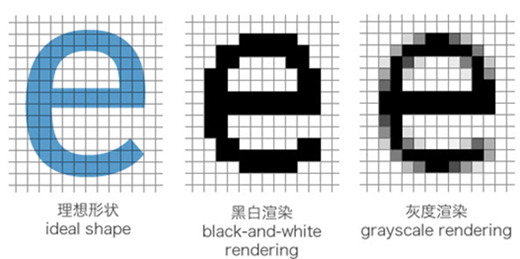##### 网站与APP开发中的字体设置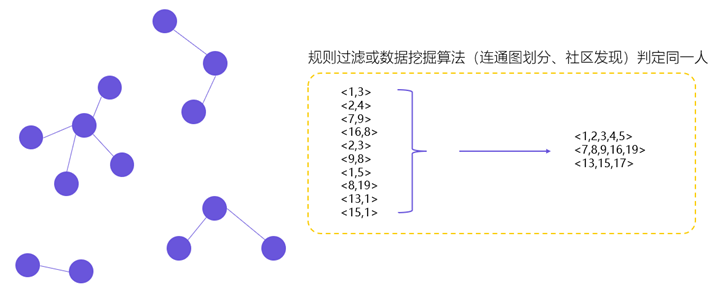##### 用户体系搭建之ID-Mapping